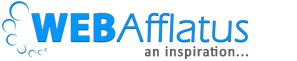# [Solved] R code for AdStock rate in Marketing mix modelingMarketing mix is an important tool to understand the effect of our marketing budget and helps to prioritize different channels. In the role of data analyst you would certainly come across this term and sometimes you would need to develop a model for the same. As we discussed in our previous blog, AdStock is one of the most important entities in Marketing mix modeling and this should be addressed while developing the model. Today I am going to write R code for the same. I want to request you to read my previous article to understand the concept.

From the previous example we can see that the effectiveness of any ad decays with time. The decay rate depends totally on business rules. The decay rate will be higher if frequency of ad is lesser and vice versa. So the decay rate depends on length of the ad, frequency and number of impressions. Frequency may be very high but customers are not watching that particular channel. So impressions will be lesser. There might be other factors as well. After discussion we can find the decay rate. Generally the effect of any ad becomes 0 after 2-3 weeks. So we will consider a window of 2 weeks only.
Let’s start the code.
From the understanding of the previous example, in my code I will consider effect rate which is vice versa of decay rate. If decay rate is 0.8 for the 2nd week then the effect rate of previous week’s ad on 2nd week will be 0.2.
Now there is inbuilt function “Filter” in R which can be used. Read more about the filter function here. In that case, only one week’s effect can be carried forward but we want to consider 2 weeks. According to our formula;
A(t) = X(t) + AdStock Rate_Week1 * A(t-1) + AdStock Rate_Week2 * A (t-2)
where,
A(t) = Cumulative effect
X(t) = Base effect
A(t-1) = Previous time period effect (last week)
A(t-2) = Last 2nd week’s effect
AdStock Rate_week1 = last 1st week’s effect
AdStock Rate_week2 = last 2nd week’s effect
So we can’t use filter function directly here. We will use our own function. Let’s create a data-set with number of impression in each week.

```abc <- data.frame(1:6)
abc\$b <- 0
colnames(abc) <- c ("Weekly impression","Effect")
```

Now let’s apply our function to calculate ad impression on 2nd,3rd and so on weeks.

```rate1 = 0.5
rate2 = 0.2
for (i in 1:nrow(abc)){
if (i ==1)
abc[i,2] <- abc[i,1]
else if (i == 2)
#Effect = impression + last week effect * decay rate
abc[i,2] <- abc[i,1] + (abc[i-1,2] * rate1)
else
#Effect = impression + last week effect * decay rate
abc[i,2] <- abc[i,1] + (abc[i-1,2] * rate1) + (abc[i-2,2]*rate2)
}

```

If we apply “Filter” function the code will be like:

```abc\$effect2 <- filter(abc\$Data1, filter = 0.5, method = "recursive")
```

Now let’s compare our output.As you can see “Filter” is considering only last week’s effect but we need to consider last 2 weeks’ effect as per our business requirement. Our job doesn’t end here. We need to make decay rate (effect rate) very carefully as different promotion activity would have different decay rate. For example TV ad will last longer than email ad.

##### Signature

Deepesh Singh## 5 thoughts on “[Solved] R code for AdStock rate in Marketing mix modeling”

1.Gabriel Leão

When i applied
> abc\$effect2 <- filter(abc\$Data1, filter = 0.5, method = "recursive")
Error in ts(x) : 'ts' object must have one or more observations

Can you say me why? 😦

•Deepesh Singh

Hi, Gabriel, the first thought that striking my mind is “filter = 0.5”. Here filter defines like AR or MA values means how many lag. So it should be an integer. Please try integer value and try again.

2.Anonymous

Try this
abc\$effect2 <- filter(abc\$"Weekly impression", filter = 0.5, method = "recursive")

3.Tomasz Kubicki

Actually you can use filter() as it allows you to pass vector of filter coefficients, not only a single one. You get the same effect as with your ‘for’ loop with “`abc\$effect <- filter(abc\$`Weekly impression`, filter = c(0.5, 0.2), method = "recursive")“`.

4.TK

Actually you can use filter() as it allows you to pass a vector of filter coefficients, not only a single one. You get the same results as your ‘for’ loop with “abc\$effect <- filter(abc\$`Weekly impression`, filter = c(0.5, 0.2), method = "recursive")".